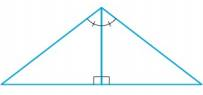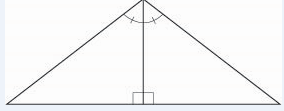Chapter A, Problem 1E### Algebra and Trigonometry (MindTap ...

4th Edition
James Stewart + 2 others
ISBN: 9781305071742

#### Solutions

Chapter
Section### Algebra and Trigonometry (MindTap ...

4th Edition
James Stewart + 2 others
ISBN: 9781305071742
Textbook Problem

# 1-4 Congruent triangles? Determine whether the pair of triangles is congruent. If so, state the congruence principle you are using.To determine

Whether the pair of triangles is congruent or not.

Solution:

The given pair of triangle is congruent by ASA.

Explanation

Given:

The given pair of triangle is,Figure (1)

Approach:

The congruence principle used is,

Angle-Side-Angle (ASA): If two angles and the included side in one triangle are congruent to the corresponding angles and side in another triangle, then the triangles are congruent.

Calculation:

The given pair of triangle is congruent by ASA principle of congruency.

If two angles and the included side in one triangle are congruent to the corresponding angles and side in another triangle, then the triangles are congruent.

Conclusion:

Hence, the given pair of triangle is congruent by ASA.

#### The Solution to Your Study Problems

Bartleby provides explanations to thousands of textbook problems written by our experts, many with advanced degrees!

Get Started